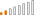cancel
Showing results for
Did you mean:Continued Contributor

## Data from database showing as decimals cannot change to whole number w/out breaking q/folding

Numeric data that is coming from my database is automatically showing as decimal numbers when I try to change it to a whole number the query folding break. Also in my database those fields are shown as integers and not decimals. Problem is when I go on power bi desktop and create a table all the numbers show with decimal points even id's. Which I then need to manually change.

1 ACCEPTED SOLUTIONSuper User

To preserve query folding, you can add a custom column with Int64.Type and use this custom column in your calculations. See below, how I am inserting one custom column by rounding the number and converting the column to Int64.Type

``= Table.AddColumn(#"Previous Step", "Custom", each Number.Round([Unit_Price],0), Int64.Type)``

Don't use below one which uses Int64.From as it does break query folding

``= Table.AddColumn(#"Previous Step", "Custom", each Int64.From([Unit_Price]), Int64.Type)``

Note - Both Number.Round and Int64.From use default rounding mode of RoundingMode.ToEven. This is what you get when you transform your column type to Whole number. Hence Number.Round to 0 decimal place yields the same result what you get from Int64.From or by selecting column and transforming column type to Whole number. Number.Round has added advantage of not breaking your query folding.

So, Rounding number to 0 decimal works whereas Int64.From doesn't work as regards query folding.Super User

To preserve query folding, you can add a custom column with Int64.Type and use this custom column in your calculations. See below, how I am inserting one custom column by rounding the number and converting the column to Int64.Type

``= Table.AddColumn(#"Previous Step", "Custom", each Number.Round([Unit_Price],0), Int64.Type)``

Don't use below one which uses Int64.From as it does break query folding

``= Table.AddColumn(#"Previous Step", "Custom", each Int64.From([Unit_Price]), Int64.Type)``

Note - Both Number.Round and Int64.From use default rounding mode of RoundingMode.ToEven. This is what you get when you transform your column type to Whole number. Hence Number.Round to 0 decimal place yields the same result what you get from Int64.From or by selecting column and transforming column type to Whole number. Number.Round has added advantage of not breaking your query folding.

So, Rounding number to 0 decimal works whereas Int64.From doesn't work as regards query folding.